#### 注册表操控(RegistryKey类搬运) 公开

| 0 | (0) | 15

##### 更多信息
 分享人 瞑空凌 分享时间 2023-06-07 15:03 最后更新 2023-07-25 16:36 修订版本 4 子程序大小 37.5 KB Quicker版本 1.38.35.0

#### 介绍

https://www.jb51.net/article/247960.htmhttps://www.jb51.net/article/247951.htmhttps://learn.microsoft.com/zh-cn/dotnet/api/microsoft.win32.registrykey?view=net-7.0参考以上毕竟是搬运的,所以为了方便,输入输出的的参数可见判定均按指定词汇,方便不同操作共用参数.毕竟搬运的应该还没有一个功能用多个相同类型参数的.{操作}(输入参数)[输出参数]----参数用类型名指定,参数可见表达式挂钩到参数类型上,方便使用.因此只需要复制黏贴,表格组合,替换,即可搞好选择列表即可直接使用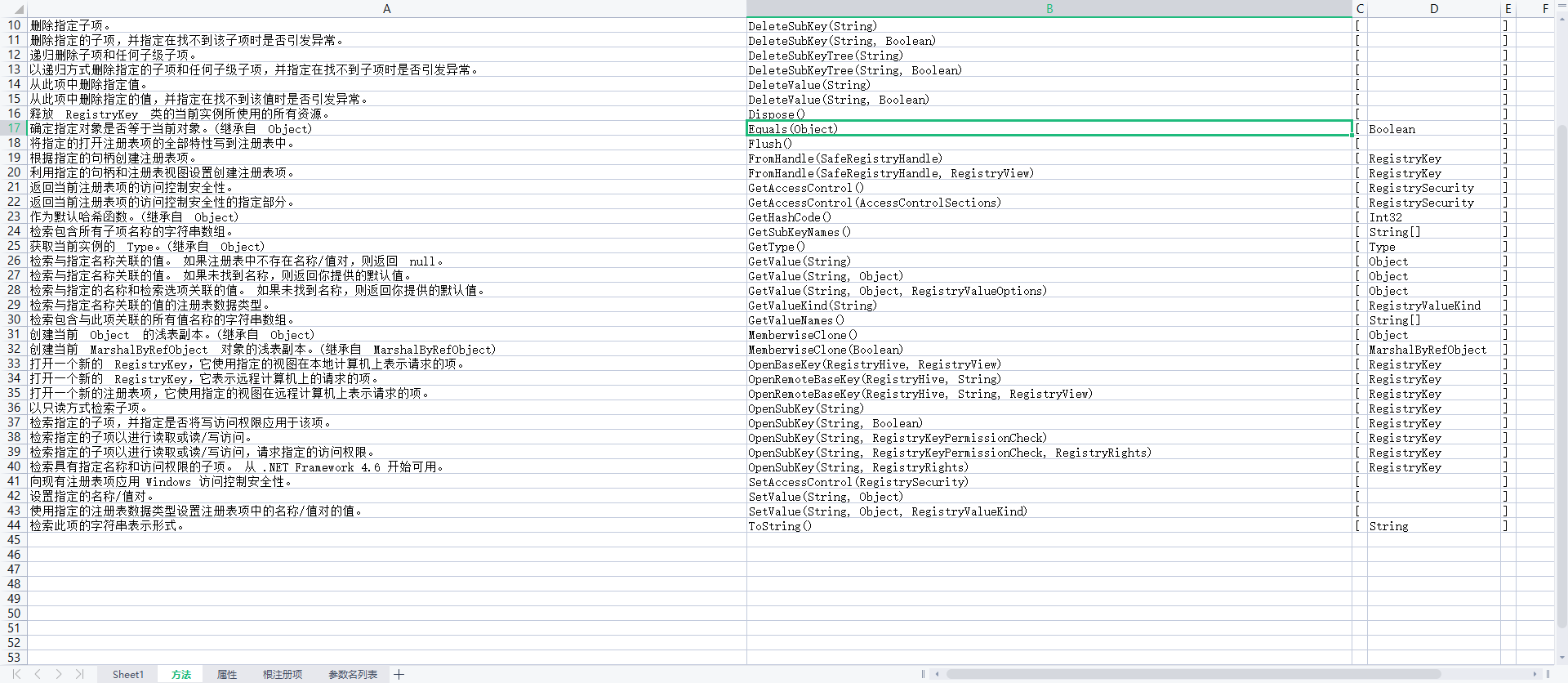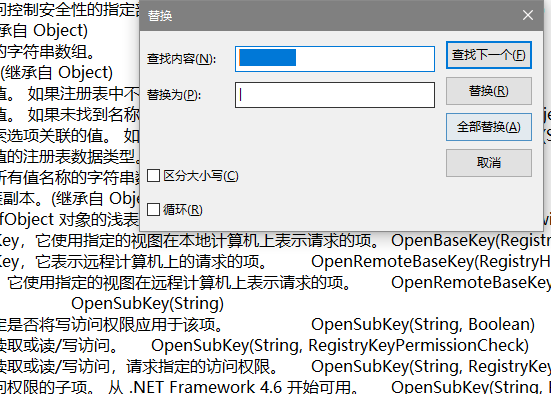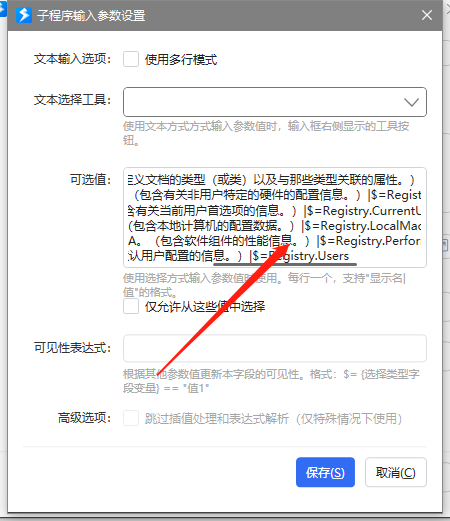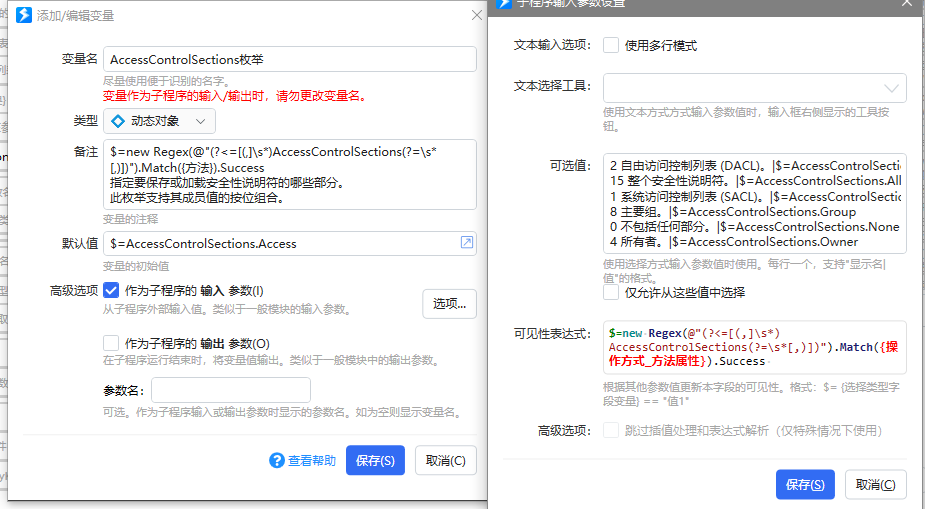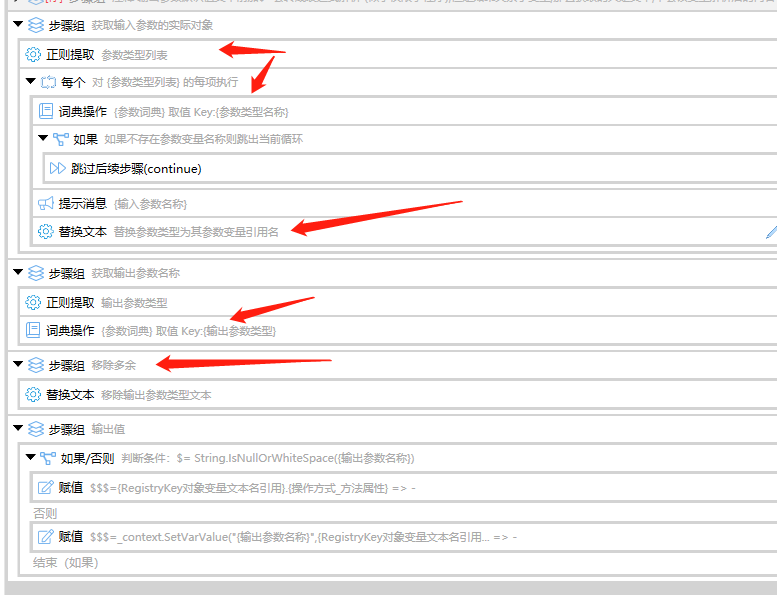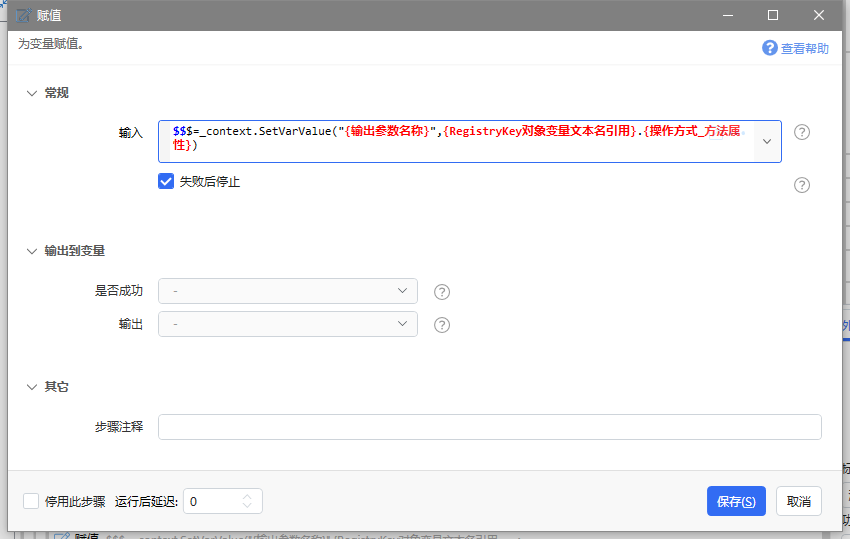#### 子程序的参数

##### 输入
RegistryKey对象 此类提供在运行 Windows 的计算机上的注册表中找到的标准根项集。 由 Registry 类公开的基或根 RegistryKey 实例描绘注册表中的子项和值的基本存储机制。 所有的项都是只读的，因为注册表依赖于它们的存在。 读取HKEY_DYN_DATA。|已过时。包含动态注册表数据。|DynData Any

String文本 \$=new Regex(@"(?<=[(,]\s*)String(?=\s*[,)])").Match({方法}).Success Text
Boolean布尔 \$=new Regex(@"(?<=[(,]\s*)Boolean(?=\s*[,)])").Match({方法}).Success Boolean
Object对象 \$=new Regex(@"(?<=[(,]\s*)Object(?=\s*[,)])").Match({方法}).Success Any
Type类型 \$=new Regex(@"(?<=[(,]\s*)Type(?=\s*[,)])").Match({方法}).Success Any
RegistryKeyPermissionCheck枚举 \$=new Regex(@"(?<=[(,]\s*)RegistryKeyPermissionCheck(?=\s*[,)])").Match({方法}).Success 指定在打开注册表项并访问它们的名称/值对时是否执行安全检查。 Any
RegistryOptions枚举 \$=new Regex(@"(?<=[(,]\s*)RegistryOptions(?=\s*[,)])").Match({方法}).Success 指定在创建注册表项时使用的选项。 此枚举支持其成员值的按位组合。 Any
RegistrySecurity类 \$=new Regex(@"(?<=[(,]\s*)RegistrySecurity(?=\s*[,)])").Match({方法}).Success 表示注册表项的 Windows 访问控制安全性。 此类不能被继承。 Any
SafeRegistryHandle类 \$=new Regex(@"(?<=[(,]\s*)SafeRegistryHandle(?=\s*[,)])").Match({方法}).Success 表示 Windows 注册表中的安全句柄。 Any
RegistryView枚举 \$=new Regex(@"(?<=[(,]\s*)RegistryView(?=\s*[,)])").Match({方法}).Success 指定要在 64 位操作系统上针对的注册表视图。 Any
AccessControlSections枚举 \$=new Regex(@"(?<=[(,]\s*)AccessControlSections(?=\s*[,)])").Match({方法}).Success 指定要保存或加载安全性说明符的哪些部分。 此枚举支持其成员值的按位组合。 Any
RegistryValueOptions枚举 \$=new Regex(@"(?<=[(,]\s*)RegistryValueOptions(?=\s*[,)])").Match({方法}).Success 指定从注册表项检索名称/值对时的可选行为。 此枚举支持其成员值的按位组合。 Any
RegistryHive枚举 \$=new Regex(@"(?<=[(,]\s*)RegistryHive(?=\s*[,)])").Match({方法}).Success 表示外部计算机上的顶级节点的可能值 Any
RegistryRights枚举 \$=new Regex(@"(?<=[(,]\s*)RegistryRights(?=\s*[,)])").Match({方法}).Success 指定能够应用于注册表对象的访问控制权限。 此枚举支持其成员值的按位组合 Any
RegistryValueKind枚举 \$=new Regex(@"(?<=[(,]\s*)RegistryValueKind(?=\s*[,)])").Match({方法}).Success 指定在注册表中存储值时所用的数据类型，或标识注册表中某个值的数据类型。 Any
##### 输出
RegistryKey对象 此类提供在运行 Windows 的计算机上的注册表中找到的标准根项集。 由 Registry 类公开的基或根 RegistryKey 实例描绘注册表中的子项和值的基本存储机制。 所有的项都是只读的，因为注册表依赖于它们的存在。 读取HKEY_DYN_DATA。|已过时。包含动态注册表数据。|DynData Any
String文本 \$=new Regex(@"(?<=[(,]\s*)String(?=\s*[,)])").Match({方法}).Success Text
Boolean布尔 \$=new Regex(@"(?<=[(,]\s*)Boolean(?=\s*[,)])").Match({方法}).Success Boolean
Object对象 \$=new Regex(@"(?<=[(,]\s*)Object(?=\s*[,)])").Match({方法}).Success Any
Type类型 \$=new Regex(@"(?<=[(,]\s*)Type(?=\s*[,)])").Match({方法}).Success Any
RegistrySecurity类 \$=new Regex(@"(?<=[(,]\s*)RegistrySecurity(?=\s*[,)])").Match({方法}).Success 表示注册表项的 Windows 访问控制安全性。 此类不能被继承。 Any
SafeRegistryHandle类 \$=new Regex(@"(?<=[(,]\s*)SafeRegistryHandle(?=\s*[,)])").Match({方法}).Success 表示 Windows 注册表中的安全句柄。 Any
RegistryView枚举 \$=new Regex(@"(?<=[(,]\s*)RegistryView(?=\s*[,)])").Match({方法}).Success 指定要在 64 位操作系统上针对的注册表视图。 Any
RegistryValueKind枚举 \$=new Regex(@"(?<=[(,]\s*)RegistryValueKind(?=\s*[,)])").Match({方法}).Success 指定在注册表中存储值时所用的数据类型，或标识注册表中某个值的数据类型。 Any
Int32整数 \$=new Regex(@"(?<=[\[]\s*)Int32(?=\s*[\]])").Match({操作方式_方法属性}).Success 表示 32 位带符号整数。 Integer
ObjRef类 \$=new Regex(@"(?<=[\[]\s*)ObjRef(?=\s*[\]])").Match({操作方式_方法属性}).Success 存储生成代理以便与远程对象通信所需的所有相关信息。 \$=//[注释]使用请参考(请直接输入对象)https://learn.microsoft.com/zh-cn/dotnet/api/system.runtime.remoting.objref?view=netframework-4.8.1 Any
StringList列表 \$=new Regex(@"(?<=[\[]\s*)String\[\](?=\s*[\]])").Match({操作方式_方法属性}).Success String[]：包含当前项的子项名称的字符串数组。 List
MarshalByRefObject类 \$=new Regex(@"(?<=[\[]\s*)MarshalByRefObject(?=\s*[\]])").Match({操作方式_方法属性}).Success 在支持远程处理的应用程序中，允许跨应用程序域边界访问对象。 \$=//[注释]使用请参考(请直接输入对象)https://learn.microsoft.com/zh-cn/dotnet/api/system.marshalbyrefobject?view=net-7.0 Any

#### 最近更新

4 2023-07-25 16:36 移除的\w+改成\w*,忘了没有输出的情况下,导致移除不干净.
3 2023-06-17 18:40 正则表达式忘了考虑有的参数类型文本带数字，因此正则参数匹配改为\w
2 2023-06-09 20:42 加入了,如果在表达式运行失败,则切换到C#模块中运行